# FI CdComp Elements

## FI CdComp General Description

The FI CdComp element is typically used to model flow through a circular hole in turbomachinery applications. This element is similar to a conventional orifice except that the Cd (Compressible Loss Coefficient) is calculated based on the geometry and flow. “CdComp” refers to the Cd being computed and “FI” is used to identify this element from a previous version of the CdComp element that is no longer recommended.

The Cd is calculated based on:
1. Reynolds Number of the flow through the hole
2. Hole corner fillet or chamfer
3. Orifice length over diameter (L/D)
4. Ratio of Pressure upstream and downstream of the hole
5. Pumping to account for hole angle and rotation
6. Incidence Angle of the flow approaching the hole
7. Difference between element and test upstream pressure

The calculation of the Cd is based on the method summarized in the paper by Idris et al. (ref 1.). A typical application would be for flow going through a hole in a compressor or turbine disk of a turbomachine. The incidence angle effect (#6 above) will account for the difference in the air and hole rotational speed. This element can be used for a wide range of applications where the circular hole Cd is not known. However, for an orifice plate in a nonrotating pipe user should consider the “Orifice Plate” element.

The element is typically used for compressible flow but Flow Simulator can use this element with incompressible or compressible fluid.

## Quick Guide for CdComp Creation in the GUI

FI CdComp can be found under the “Compressible Gas Elements” or “Incompressible Liquid Elements” - “Orifices and Generic Losses” section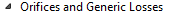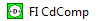The correct “Part Surface Angle” and “Element Orientation” are critical for an accurate flow incidence angle. One thing to remember is that if the hole centerline is perpendicular to the part surface the “Part Surface Angle” and “Element Orientation” are the same. The “Part Surface Angle” and “Element Orientation” will only be different if the hole is at an angle to the part surface. Examples of “Part Surface Angle” and “Element Orientation” are shown below.

## FI CDComp Inputs

Table of the inputs for the Cd comp element. See the image in the user interface to help understand geometric variables.

FI CDComp Element Input Variables
Index UI Name (.flo label) Description
1 Area (AREA) Orifice mechanical area. A diameter can also be entered in the UI and an area based on a circular hole is calculated.
2 Not in UI (K_INLET) Not used in solver
3 Not in UI (EXIT_XFLOW) Not used in solver
4 Heat Addition Mode (HEAT_MODE) Mode of heat transfer to/from the fluid in the element.

5 Not in UI (ROUGHNESS) Not used in solver

Or

Chamfer Length (CORNER_R)

When Corner Type (CORNTYP) is 3.0 (Chamfer), this field becomes the Orifice chamfer length (in) and is used in conjunction with the Chamfer Angle (CHAM_ANG) to describe the inlet geometry.

7 Length (LENGTH) Orifice length
8 Hydraulic Diameter (HYD_DIA) Orifice hydraulic diameter
9 Reverse Flow Method (REV_METHOD) The Cd calculation method to use if the flow reverses in this orifice. This may be required if the corner treatment is different on the two ends of the hole.

0: Same as forward; use same corner treatment as forward flow.

1: Different inputs; the corner treatment is different for forward and reverse flow.

3: Fixed Cd; use a fixed Cd for reversed flow.

10 Fixed Cd (CD_REV) The Cd to use for reversed flow if REV_METHOD=3 (Fixed Cd)
11 Element Inlet Orientation: Tangential Angle (THETA) Angle between the element centerline at the entrance of the element and the reference direction.

If the element is rotating or directly connected to one or more rotating elements, the reference direction is defined as parallel to the engine centerline and the angle is the projected angle in the tangential direction. Otherwise, the reference direction is arbitrary but assumed to be the same as the reference direction for all other elements attached to the upstream chamber.

Theta for an element downstream of a plenum chamber has no impact on the solution.

12 Element Inlet Orientation: Radial Angle (PHI) Angle between the element centerline at the entrance of the element and the THETA direction (spherical coordinate system).

Phi for an element downstream of a plenum chamber has no impact on the solution.

13

14

15

Exit K Loss:

Axial (K_EXIT_Z)

Tangential (K_EXIT_U)

Head loss factors in the Z, U, and R directions, based on the spherical coordinate system of theta and phi.

Z theta and phi equal zero (the axial direction in a rotational field).

U theta equals 90 and phi equals zero (the tangential direction in a rotational field).

R theta equals zero and phi equals 90 zero (the radial direction in a rotational field).

Valid values of K_EXIT_i (i = Z, U, R) range from zero (default) to one

The three loss factors reduce the corresponding three components of velocity (idir) exiting the element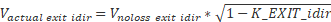Default value, 0.0, provides no loss.

16 Portion of UStrm Cham. Dyn. Head Lost (DQ_IN) Inlet dynamic head loss. Valid range is 0.0 to 1.0 inclusive. An entry outside this range causes a warning message and the value used will be 0 or 1 (Whichever value is closest to the entry).

If DQ_IN > 0 and the upstream chamber has a positive component of relative velocity aligned with the centerline of the orifice, the driving pressure is reduced by the equation: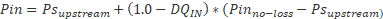Default value = 0.

17 Inlet Radius (RIN) Radius (in). Radial distance between the orifice inlet center and the engine centerline.

Do not use zero unless the orifice is not rotating.

18 Corner Type (CORNTYP) Orifice entrance corner type.

1.0: Sharp entrance (default)

2.0: Filleted entrance equation

3.0: Chamfered entrance equation

CORNTYP=0.0 is corrected to CORNTYP=1.0 by both the Flow Simulator Solver and GUI.

19 Rotor Index (RPMSEL) Element rotational speed pointer.

0.0: Specifies a stationary element.

1.0: Points to general data ELERPM(1).

2.0: Points to general data ELERPM(2).

3.0: Points to general data ELERPM(3).

-1.0: Element RPM is based on upstream fluid RPM.

20 Heat Input (QIN) The value entered for QIN depends on the HEAT_MODE chosen.

22 Chamfer Angle (CHAM_ANG) Angle between corner chamfer and the hole centerline. Only used when CORNTYP=3.0.

Default is 0.0.

23 Part Surface Angle: Tangential (THETA_SNORM) Part surface normal vector tangential angle (degrees).

*Uses the same angle convention as the orifice itself. For example, if the hole is drilled normal to the part surface, the THETA_SNORM should be set equal to THETA)

Default is 0.0.

24 Part Surface Angle: Radial (PHI_SNORM) Part surface normal vector radial angle .
25 Swirl Carryover (SWLCO) The amount of relative tangential velocity in the upstream chamber to carry over to the downstream chamber.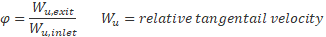If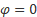, no swirl is carried over and the air exits the hole at wheel speed (swirl = 1); swirl carryover is near 0 for holes with any significant length (L/D > 0.3)

26 Flow Equation Type (INCOMPR_FLG) Flag to specify flow compressibility for element calculations (Compressible vs. Incompressible).

This is not actually used by the solver. The solver automatically changes equations based on the phase of the fluid entering the element. The element uses compressible equations for a gas and incompressible equations for a liquid.

27 Reverse Corner Type (REV_CORNTYP) The type of corner treatment to be used for reverse flow. Only used if REV_METHOD=1

See CORNTYP

28 Corner Radius (REV_CORNER_R) The corner radius or chamfer length for reverse flow. Only used if REV_METHOD=1.

See CORNER_R.

29 Reverse Chamfer Angle (REV_CORNER_ANG) The corner chamfer angle for reverse flow.

Only used if REV_METHOD=1.

See CORNER_ANG.

30 Fraction of QIN applied before restriction (QIN_RATIO) Fraction of total heat to be added before the restriction. (0 = None, 1 = All)

31 Exit Radius (REX) Radius (in). Radial distance between the orifice exit center and the engine centerline. This radius is used for pumping calculations for a rotating hole.

32 Reynolds Number (RE_METHOD) Equation to use for the Reynolds number portion of the Cd calculation.

0: McGreehan (default)

1: McGreehan Nozzle

>1000: A custom equation

33 Corner (CORNER_METH) Equation to use for the Corner type portion of the Cd calculation. Default is based on CORNTYP

-1: No Corner modification used if CORNTYP=1

0: McGreehan Fillet used if CORNTYP=2

1: Idris Chamfer used if CORNTYP=3

>1000: A custom equation

34 Length (LD_METHOD) Equation to use for the Hole Length portion of the Cd calculation.

-1: None

0: Huning (default)

>1000: A custom equation

35 Pressure Ratio (PR_METHOD) Equation to use for the Pressure Ratio portion of the Cd calculation.

-1: None

0: Idris (default)

>1000: A custom equation

36 Pumping (PUMP_METHOD) Equation to use for the Pumping portion of the Cd calculation. This is not for the pressure and temperature change due to rotation.

-1: None

0: Idris (default)

>1000: A custom equation

37 Incidence Angle (ANG_METHOD) Equation to use for the Incidence Angle portion of the Cd calculation.

-1: None

0: Idris (default)

1: Huning

2: Weisbach (angled hole with no inlet velocity)

>1000: A custom equation

## FI CdComp Theory

Flow Rate Calculation

The compressible flowrate is calculated using the following “flow function” equation (ref 5):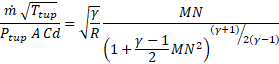The mach number (MN) is related to the pressure ratio using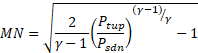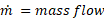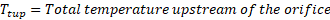(aka TTS)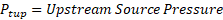(aka PTS)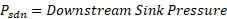(aka PSEB)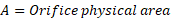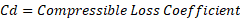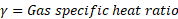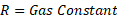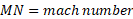Other references (1,2) also have the flow function equation in different forms but they all represent the same thing.

The incompressible flowrate is calculated using the following: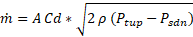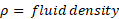Cd Calculation

The calculation of the Cd is based on the method summarized in the paper by Idris et al. (ref 1.). The method steps though several characteristics of the orifice and the flow to calculate the final Cd. The details of each step are shown below.

Reynolds Number Effect

The first step is the related to the hole Reynolds number (Re). The Reynolds number is based on the “ideal” velocity through the hole.

For an orifice: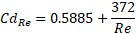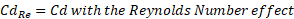For a nozzle:

$C{d}_{Re}=.9981-\frac{4.73}{\sqrt{Re}}$

The Cd is not very sensitive to the Re. The minimum Re used in this equation is 10,000. The Cd used as the basis for the next step is always around 0.6 for an orifice.

Hole Corner Fillet or Chamfer

A fillet or chamfer can significantly reduce the losses in the hole (a higher Cd).

For a hole with a circular fillet (McGreehan Fillet, ref 1):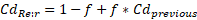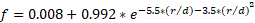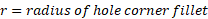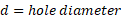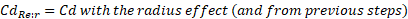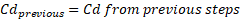The example shows streamlines entering a hole with a sharp corner and a hole with a large fillet. The hole with a sharp corner shows flow separation while the hole with a radius does not. The plot demonstrates the radius effect and may not represent the final Cd.

For a hole with a chamfer (Idris Chamfer, ref 1):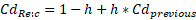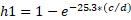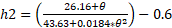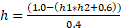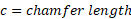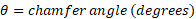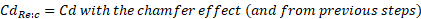The plot demonstrates the radius effect and may not represent the final Cd.

Orifice Length over Diameter (L/D)

The length of the orifice affects the reattachment of the orifice flow. If the flow can reattach inside the orifice length the losses can be reduced (Cd increased). The program adjusts the length due to a fillet or chamfer so the user should enter the orifice length as if there was no fillet or chamfer. The program does not adjust the length for the hole angle. The user should enter a hole length that includes the angle effect. Equation taken from Huning, ref. 2.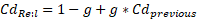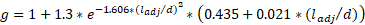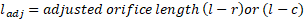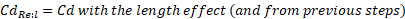The plot demonstrates the length effect and may not represent the final Cd. Note that the maximum Cd occurs between L/D = 1.5 and 2. Friction losses increase as the orifice gets longer so Cd decreases for L/D > 2

Ratio of Pressure Upstream and Downstream of the Hole

The pressure ratio effect accounts for the compressibility of the gas. It will have no affect for liquid (incompressible) fluids. The maximum pressure ratio used in this equation is 5.0. As the pressure ratio increases the vena contracta increases to cause the higher Cd. Equation taken from Idris, ref. 1.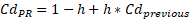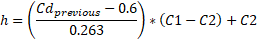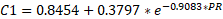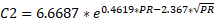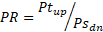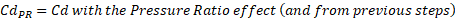The plot demonstrates the pressure ratio effect and may not represent the final Cd. Note that the Cd increases after the choking pressure ratio (~1.9). So, the orifice flowrate may increase after the PR exceeds the choking PR.

Pumping to Account for Hole Angle and Rotation

Idris (ref 1) describes a pumping effect that accounts for the fact that the Cd for Incidence angle = 0o is higher for a rotating hole (which must be angled to achieve zero incidence) that it is for a stationary hole. The physics behind this are not clear. The effect is included to match the Idris data, ref. 1.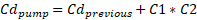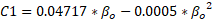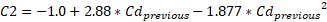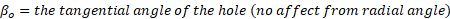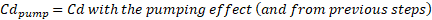The plot demonstrates the pumping effect and may not represent the final Cd.

Incidence Angle of the Flow Approaching the Hole

The final Cd is very sensitive to the incidence angle effect. If the upstream flow approaches a hole at a steep angle a large separation region can fill a significant portion of the hole. This will decrease the Cd and flowrate. The incidence angle affects are taken from ref 1. The hole corner treatment and the angle of the hole influence the incidence angle effect.

For holes that are perpendicular to the part surface:

For a hole with a sharp corner (Idris):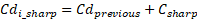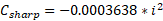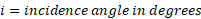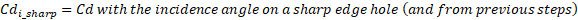The plot demonstrates the incidence effect and may not represent the final Cd. The absolute value of the incidence angle is used in this equation. The maximum incidence angle allowed is 45o.

For a hole with a circular fillet (Idris):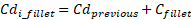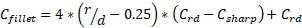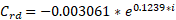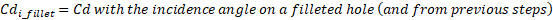The plot demonstrates the incidence effect and may not represent the final Cd. The absolute value of the incidence angle is used in this equation. The maximum incidence angle allowed is 45o. The maximum r/d allowed is 0.25 .

For a hole with a chamfer (Idris):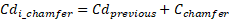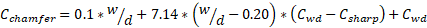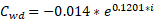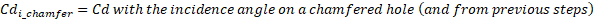The plot demonstrates the incidence effect and may not represent the final Cd. The absolute value of the incidence angle is used in this equation. The maximum incidence angle allowed is 30o. The maximum c/d allowed is 0.20.

There is also an equation from Huning (ref 2) for holes that are perpendicular to the surface. The same equation is used for all corner types (sharp, fillet, and chamfer).

$C{d}_{i}=C{d}_{previous}*Hunin{g}_{mult}$

$Hunin{g}_{mult}=-.00044513*{i}^{2}-.0029937*i+1.0$

For holes that are angled to the part surface:

There is no data available for the incidence angle effect on an angled hole with a fillet or chamfer. The sharp corner Cd will be used even if the hole has a fillet or chamfer. The effect is different for holes with positive and negative incidence.

For a hole with a sharp corner (Positive incidence) :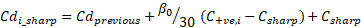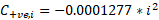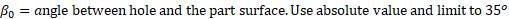For a hole with a sharp corner (Negative incidence) :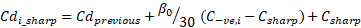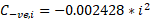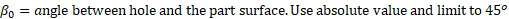The following plots show the effect of incidence angle on holes that have a 15o, 30o, and 45o angle with the part surface. The plots demonstrate the incidence effect and may not represent the final Cd.

The Weisbach adjustment for an angled hole is valid when the upstream velocity is 0, in other words, a plenum chamber is upstream of the Cdcomp element. It is based on the following equation from Weisbach, but can also be found in Ref 9.

$K=0.5+0.3\mathrm{cos}\left(i\right)+0.2{\text{cos}}^{2}\left(i\right)$

The K is converted into a Cd using Cd=1/sqrt(1+K) to get an adjustment to the previous Cd.

Difference between Element and Test Upstream Pressure

The final effect is not from the Idris paper and is specific to Flow Simulator. The empirical based Cd equations and Flow Simulator may use different upstream pressures (Ptup, aka PTS) in the flow function calculation. The test typically used a total pressure based on the relative velocity but Flow Simulator typically uses a pressure less than this total pressure. The pressure that Flow Simulator uses is based on the upstream chamber velocity vector that is aligned with the centerline of the hole, and the entrance loss setting (Portion of Ustrm Cham. Dyn. Head Lost).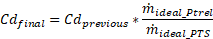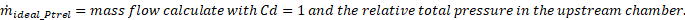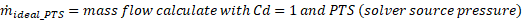Reverse Flow

The Cd calculated for reversed flow may be different than the Cd calculated for forward flow due to different corner treatment or other flow changes. One end of the hole is filleted and the other is sharp, for instance. The Cd for a reversed flow can be calculated using the same inputs as for forward flow, or the corner treatment can be adjusted. A fixed Cd can also be used.

## FI CDComp Outputs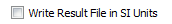Outputs in file with RES extension. Output units controlled by user setting in “Output Control” panel.

Name Description Units ENG, SI
ELEMENT_AREA Cross-sectional area.

Usually, an echo of the user input unless modified inside Flow Simulator.

inch2, m2
CD Final discharge coefficient.

Calculated in a multi-step process in the CDCOMP routine.

(unitless)

Usually, an echo of the CORNER_R input unless modified inside Flow Simulator.

Inch, mm
L Length.

Usually, an echo of the LENGTH input unless modified inside Flow Simulator.

Inch, mm
D Hydraulic diameter.

Usually, an echo of the HYD_DIA input unless modified inside Flow Simulator.

Inch, mm
RPM Rotational speed of the restriction rev/min
RIN Radius to the orifice inlet from the engine centerline.

Usually, an echo of the RIN input unless modified inside Flow Simulator.

Inch, m
THETA_SNORM Part surface normal vector tangential angle degrees
PHI_SNORM Part surface normal vector radial angle (degrees). degrees
PTS Driving pressure relative to the rotational reference frame (that is, rotor) at the restriction inlet. Psia, MPa
PTEX Total pressure relative to the rotational reference frame (that is, rotor) at the restriction exit including supersonic effects. Psia, MPa
PSEX Static pressure relative to the rotational reference frame (that is, rotor) at the restriction exit.

Limited by critical pressure ratio for supersonic flows.

Psia, MPa
PSEB Effective sink (static) pressure downstream of the restriction. Psia, MPa
TTS Total relative temperature of fluid entering the element deg F, deg K
VCMN Fluid Mach number relative to the rotational reference frame (that is, rotor) at the vena contracta. (unitless)
VXA Fluid velocity relative to the rotational reference frame (that is, rotor) at the restriction exit before heat input (QIN) effects. ft/s, m/s
EXMN Fluid Mach number relative to the rotational reference frame (that is, rotor) at the restriction exit before heat input (QIN) effects. (unitless)
QIN Heat input.

Positive values indicate heat added to the fluid; negative values indicate heat removed.

BTU/s, W
DT Change in total temperature relative to the rotational reference frame (that is, rotor) due to heat input (QIN). deg F, deg K
TEX Total temperature relative to the rotational reference frame (that is, rotor) at the restriction exit. deg F, deg K
VEX Fluid velocity relative to the rotational reference frame (that is, rotor) at the restriction exit including heat input (QIN) effects. ft/sec, m/s
CDRE Incompressible discharge coefficient based on the fluid Reynolds number. (unitless)
CDR Incompressible discharge coefficient (CDRE) adjusted for inlet corner radius effects. (unitless)
CDRL Incompressible discharge coefficient (CDR) adjusted for effects of length or thickness. (unitless)
CDRL_PR Incompressible discharge coefficient (CDRL) adjusted for compressibility effects. (unitless)
CDRL_PR_PUMP Compressible discharge coefficient (CDRL_PR) adjusted for hole angle and rotation. (unitless)
CDRLU Compressible discharge coefficient (CDRL_PR_PUMP) adjusted for effects of fluid rotational speed relative to the rotational reference frame (that is, rotor) at the restriction inlet. (unitless)
CD Compressible discharge coefficient (CDRLU) adjusted for effects of source pressure difference between empirical equations and Flow Simulator PTS. Same as the “Final Discharge Coefficient” already listed in the element output. (unitless)
V_IDEAL Ideal velocity in the hole.

Use Cd=1 and Ptrel as the upstream pressure.

ft/sec, m/s
SPARV_UP Total magnitude of hole upstream velocity parallel to the part surface. ft/sec, m/s
SPARV_Z Axial component of hole upstream velocity parallel to the part surface. ft/sec, m/s
SPARV_R Radial component of hole upstream velocity parallel to the part surface. ft/sec, m/s
SPARV_U Relative tangential component of hole upstream velocity parallel to the part surface. ft/sec, m/s
SWLCO Swirl Carryover through the hole (unitless)
XK_INLET Swirl Ratio upstream of the hole. (unitless)
RPM_REF_IN Reference RPM for the swirl ratio upstream of the hole. rev/min
XK_EXIT Swirl Ratio downstream of the hole. (unitless)
RPM_REF_EX Reference RPM for the swirl ratio downstream of the hole. rev/min

## FI CDComp Validation

The FI CDComp results were compared to Cd and flow results from various tests as shown below.

## CDComp References

1. A. Idris, K. R. Pullen, “Correlations for the discharge coefficient of rotating orifices based on the incidence angle”, Proceedings of IMechE, Part A: J. Power and Energy, Vol-219, Year-2005.
2. Marcus Hüning, “Comparison of Discharge Coefficient Measurements and Correlations for Orifices with Cross-Flow and Rotation”, Journal of Turbo machinery, Vol-132, p031017-1-10, Year-2010.
3. A. Alexiou, N.J. Hills, C.A. Long, A.B. Turner, L.-S. Wong, J.A. Millward, “Discharge coefficients for flow through holes normal to a rotating shaft”, International Journal of Heat and Fluid Flow, Vol-21, p701-709, Year-2000.
4. W. F. McGreehan, M. J. Schotsch, “Flow Characteristics of Long Orifices With Rotation and Corner Radiusing”, Journal of Turbo machinery, Vol-110, p213-217, Year-1988.
5. Miller, D.S., “Internal Flow Systems,” Miller Innovations, 2nd Edition, 1990.
6. Rhode, J. E., Richards, H. T., Metger, G. W., Discharge Coefficients for Thick Plate Orifices With Approach Flow Perpendicular and Inclined to the Orifice Axis, 1969, Technical Report, NASA-TN-D-5467.
7. Dittmann, M., Dullenkopf, K., Witting, S., Discharge coefficients of rotating short orifices with radiused and chamfered inlets, J. Turbomach.,2004, 126, 803-808.
8. Wittig, S., Kim, S., Jakoby, R., WeiBert, I., Experimental and Numerical Study of Orifice Discharge Coefficients in High-Speed Rotating Disks, Transactions of the ASME, 1996, 118, 400-407.
9. Blevins, R. D., Applied Fluid Dynamics Handbook, Krieger Publications, 2003.